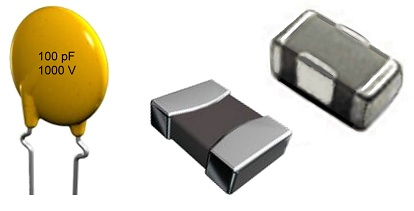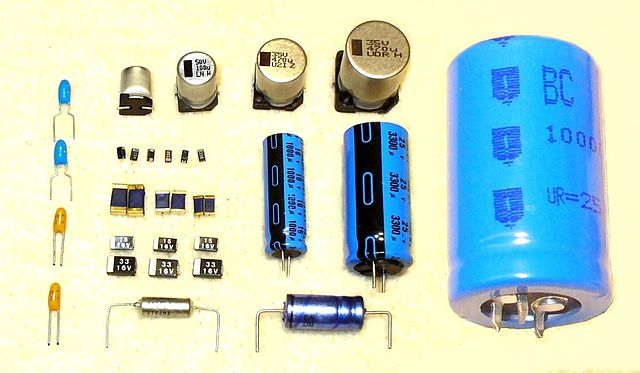# Difference Between Ceramic and Electrolytic Capacitor

## Main Difference – Ceramic vs. Electrolytic Capacitor

Ceramic and electrolytic capacitors are two types of capacitors used in electronic circuits. The main difference between ceramic and electrolytic capacitor is that, in ceramic capacitors, the two conductive plates are separated by a ceramic material whereas, in electrolytic capacitors, the two conductive plates are separated by an electrolyte and a metal oxide layer.

## Structure of a Capacitor

A capacitor is a device that can store electrical energy. Although there are different types of capacitors, most of them are based on the same basic blueprint. Taken simply, a capacitor consists of two conductive plates separated by an insulating material called the “dielectric“. The main structure is shown below:Basic structure of a capacitor

The capacitance of the capacitor describes how much charge that a capacitor stores when there is a given potential difference across it.  If each of the conductive plates has an area$A$ and they are separated by a distance$d$, then the capacitance$C$ is given by:$C=\varepsilon\frac{A}{d}$

where$\varepsilon$ is the permittivity, which is a property of the dielectric substance.

## What is a Ceramic Capacitor

A ceramic capacitor is a type of capacitor whose dielectric is a ceramic material. In the simplest construction of these, a layer of a ceramic material sits between two conductive plates. However, the most frequently-used type of ceramic capacitors is the so-called multi-layer chip capacitors (MLCC). In MLCCs, there is a number of conductive plates and a ceramic material is sandwiched between each pair of plates. Effectively, they work as though they are many small capacitors in parallel, which gives a large combined capacitance.Ceramic capacitors: single layer (left) and multilayer (right)

There are two main types of ceramic capacitors: class 1 and class 2. Class 1 capacitors are more accurate and stable over a larger range of temperatures while class 2 capacitors offer more volumetric efficiency (more capacitance per unit volume).

## What is an Electrolytic Capacitor

An electrolytic capacitor is a type of capacitor that uses an electrolyte in order to increase its capacitance. Typically aluminium, tantalum, or niobium acts as the conductive material. The dielectric in these capacitors is the oxide layer that forms on these metals. Since these oxide layers are very thin, the$d$ in the capacitance equation above is very small, making the capacitance of the capacitor very high. In the space between the conductors, there are papers which are soaked in an electrolyte. The electrolyte itself acts as the anode while one of the metal plates acts as the cathode.Some electrolytic capacitors

Electrolytic capacitors are polarized. This means that when they are connected to circuits, each terminal needs to be given the correct polarity. If they are connected with the wrong polarity, they may become very hot and may even explode. For electrolytic capacitors, the equivalent series resistance (ESR) is an important concept. If the ESR is too high, then the current flow through the circuit would be too small. The figure below shows how the ESR (impedance) of a capacitor changes with resistance. Each curve shows different capacitance values:Impedance as a function of frequency for capacitors of different capacitances

Note that for each type of capacitor, there is a frequency at which the impedance is at a minimum. This frequency is the resonant frequency of the capacitor. Note that as the capacitance becomes larger, the resonant frequency becomes smaller.

## Difference Between Ceramic and Electrolytic Capacitor

### Structure:

In ceramic capacitors, ceramics separate the conductive surfaces.

In electrolytic capacitors, metal oxide layers and an electrolyte separate the conductive surfaces.

### Dielectric:

In ceramic capacitors, a ceramic substance makes up the dielectric.

In electrolytic capacitors, the dielectric consists of a very thin oxide layer.

### Polarization:

Ceramic capacitors are not polarized.

Electrolytic capacitors are polarized.

### ESR:

Ceramic capacitors typically have low ESRs.

The ESR in electrolytic capacitors is typically higher and more strongly dependent on the frequency.

### Microphony:

Ceramic capacitors exhibit microphony: an effect where mechanical vibrations lead to electrical noise in circuits.

Electrolytic capacitors do not exhibit microphony.

Image Courtesy:

“A diagram of a simple parallel plate capacitor” by inductiveload (own drawing, done in Inkscape 0.44) [Public Domain], via Wikimedia Commons

“Keramik-Scheibenkondensator” by Elcap, Jens Both (own work) [GFDLv1.2], via Wikimedia Commons (Modified)

“Mlcc-Bauformen” by Elcap, Jens Both (own work) [GFDLv1.2], via Wikimedia Commons (Modified)

“Some different styles of aluminum and tantalum electrolytic capacitors” by Elcap (own work) [CC0 1.0], via Wikimedia Commons

“Impedanzkurven verschiedener Kapazitätswerte aus unterschiedlichen Kondensatorfamilien” by Elcap, Jens Both (own work) [GFDLv1.2], via Wikimedia Commons